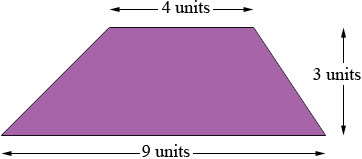SEARCH HOMEMath Central Quandaries & QueriesQuestion from kelsey, a student: The area of a trapezoid is approximately 150cm2...the length is 26 cm, the weight is 5cm...wht is the height???? (show me the work that you did so i can understand)Kelsey,

I don't understand this problem. I think "weight" must be a typo but even then it is confusing.

A trapezoid has two parallel sides and its area is

the average of the lengths of the parallel sides times the distance between the parallel sides.

Here is an exampleThe parallel sides have lengths 9 and 4 units so the average is (9 + 4)/2 units. The distance between the parallel sides is 3 units and hence the area is

[(9 + 4)/2] × 3 = 19.5 square units.

Notice that If I told you the lengths of the parallel sides were 9 and 4 cm, the area was 19.5 square cm and asked for the distance x between the parallel sides then the area expression would be

[(9 + 4)/2] × x = 19.5 square cm.

I could then solve for x to find x = 3 cm.

I hope this helps,
PennyMath Central is supported by the University of Regina and The Pacific Institute for the Mathematical Sciences.